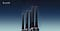# Deep Learning and the Functioning of Artificial Neural Networks

## A brief excursion into the world of Artificial Intelligence

Deep Learning is the technology in the field of Machine Learning, which has been one of the most important development steps in Artificial Intelligence in recent years. Due to the current progress and the assumed importance of Deep Learning in the future design and development of Artificial Intelligence, it was important for me to better understand the principle of Deep Learning and to share it from layman to layman ;-)Marius Goebel — Deep Learning and the Functioning of Artificial Neural Networks — Photo by Simone Hutsch

The artificial neural networks are inspired by the human brain and the neural networks it consists of. The human brain has about 100 billion neurons, each of which is connected to a multitude of other neurons. A neuron is basically a cell with many input channels for receiving incoming signals, called dendrites, and one output channel, the axon. Each neuron contains a nucleus, which can generate an electrochemical signal as soon as the incoming signals exceed the respective so-called threshold value of the neuron.

This signal in turn reaches other neurons where this process is repeated. Artificial neural networks mimic this architecture and the behaviour of neurons in our brain. Neurons in an artificial neural network can be understood as mathematical approximation functions. Each neuron holds an indefinite number of signal input channels, for each of these input channels a weight can be determined, which determines the relevance of the input signal.

Thus the contribution of each input to the output is determined by the weight assigned to each input. In addition, a threshold value can be programmed for each neuron itself, which determines at what intensity the neuron sends a signal. The artificial neuron thus transforms the input signals into a single output signal, this transformation depending on the weight of the inputs, the mathematical function and the respective threshold value (bias) of the neuron.

In contrast to the complex entanglement of the billions of neurons in the human brain, an artificial neural network consists of thousands of neurons in different layers. Deep learning nets are arranged in layers so that each layer can pass on the output of the mathematical function to the next layer. The output of the last level is the sum of the decisions of the network based on the input in the first level. The levels between the input level, the first level of the layered network and the output level, the last level (also result layer), are called hidden layers.

The input to artificial neural networks is always converted into numbers. If these numerical values exceed the threshold value of one or more neurons as described above, a signal is sent to the next layer, which in turn flows as numerical values into the signal inputs of this next layer, where they are multiplied again by the weights of the inputs. If these weighted input values again exceed the threshold value of the neurons, corresponding output values are passed on to the next layer.

Within an artificial neural network, the complexity increases per layer on the trigger of the respective neurons. For example, the neurons of a deep learning network, which was trained for image recognition, are responsible in the first layer for the recognition of corners and edges, the second layer possibly for more complex patterns, another for the recognition of human facial features, in the output layer all unimportant or wrong results are filtered out in a trained neural network, thus the output layer only represents what the network was trained for. In this example it could be the output of all images stored in the internet by David Hasselhoff.

To train an artificial neural network accordingly, it is necessary to provide the neural network with so-called labelled data. This means that the data must “know” what they themselves represent. This kind of data is expensive and time-consuming to create in comparison to unlabeled data, which are available in masses. In order to learn an untrained network, the parameters of the neurons are randomly adjusted and then millions of labelled data together with unlabelled data are fed into the neural network as data input.

Initially, output decisions will be wrong, but based on the labelled data, the results can be divided into appropriate classes and the neurons that contributed most to the wrong classes being activated can be identified. The network is trained by the feedback on the correctness or incorrectness of the result and can thus readjust the weighting of the input signals and the thresholds of the neurons that were responsible for the least false results.

If the network is trained, this means that the parameters have been set to detect and output the correct results. From this point on, unlabelled data can be started to be input to the artificial neural network.

Once a network has learned to recognize the desired output, it can independently perform what it has learned with unlabeled data and deliver the correct output. Once the learning process is complete, a Deep Learning network is even able to use this unlabeled data to independently adjust the weightings and thresholds to improve its performance. In a perfectly tuned network the output decisions are always correct independent of the input.

In simple terms, neural networks are pure function-axipromators, which attempt to approximate a function that provides the corresponding result exclusively in the area in which they were trained. As already mentioned, artificial neural networks today are only able to do one thing perfectly — for example the recognition of images — but then mostly better than humans.

Beep boop beep — Cheers ;-)

Max Tegmark: Life 3.0 — Being Human in the Age of Artificial Intelligence

Andrew McAfee, Erik Brynjolfsson: Machine, Platform, Crowd — Harnessing Our Digital Future

Written by

Written by

## Marius Goebel

#### Freelance Marketing & Digital Strategy Consultant — Creating meaningful brands — www.kontik.de